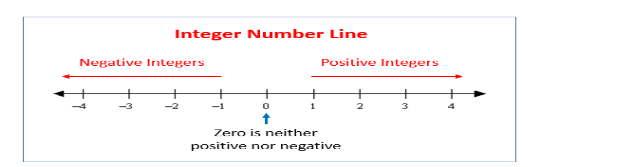QuestionAnswers

# For any integer $a$, $a+0=$ A. 1B. 2C. aD. 0

Hint: f any number is added by number 0 then it always results into that number because 0 has no value
But if we add any positive or negative number then it will increase or decrease the total sum because it has some value with it. We can better understand it using number line

Given an integer a and we have to find the value of $a+0=$
This we can understand using number lineIn number line we have all integers and here addition or subtraction means moving some distance
Rightward or leftward
Let’s take an example
Consider a number 2 and we are adding it with number 1: $2+1=3$
Then look at the 2 number in number line now we add number 1 it means it we are moving 1 unit rightward from number 2 and as shown as number line it gives result 3
Consider another example this time we add number 2 with number -3: $2+(-3)=-1$
Then look at the 2 number in number line now we add number -3 it means it we are moving 3 unit leftwards from number 2 and as shown as number line it gives result -1
Now think about 0, when we add it with any number It gives result same as that number only
Because adding number 0 means travelling distance 0 in number line which means no movement at all
So, the correct answer is “Option C”.

Note: Most of student did mistake while solving questions like adding negative number to some number or subtracting negative number to some number
For example : adding 2 with -3 means $2+(-3)$,which can be written as $2-3=-1$ meaning moving 3 distance leftward from number 2
Example: subtracting -3 from 2 means $2-(-3)$ which can be written as $2+3=5$ meaning moving 3 distance rightward from number 2